Universal Odeand#8217;s and Their Solution | OMICS International
Journal of Physical Mathematics

# Universal Ode’s and Their Solution

Cusack P*

*Corresponding Author:
Cusack P
Tel: +264854144
E-mail: [email protected]

Received Date: June 03, 2016; Accepted Date: July 26, 2016; Published Date: August 01, 2016

Citation: Cusack P (2016) Universal Ode’s and Their Solution. J Phys Math 7: 191. doi: 10.4172/2090-0902.1000191

Copyright: © 2016 Cusack P. This is an open-access article distributed under the terms of the Creative Commons Attribution License, which permits unrestricted use, distribution, and reproduction in any medium, provided the original author and source are credited.

Visit for more related articles at Journal of Physical Mathematics

#### Abstract

This paper presents a solution to the ODE’s that govern our universe. It also discusses the Mass Gap, Free Will, the Speed of Light, and the stable universe. The mathematics of time travel is presented. In addition, the solution to the Second Order DE is presented when the constants are equal

#### Keywords

ODE; Stable universe; Time travel; Second ODE; Primes; Singularity

#### Introduction

The Universal ODE is presented as well as its solution. Finally, a treatment of Prime Numbers and their significance is presented. The universe is shown to be a singularity.

#### The Distance Ordinary Differential Equation and the eigenvector Time

The Laplace transform for the second order linear differential equation is the famous distance equation in physics (Figures 1-4).

d=vit+1/2 at2

s=st+1/2 s''t2

s=1/2s''t2+st

t=1

s=1/2s''+s'

Let s'=et & multiply by (et)2

1/2 s'' (s')2 + (s')3

If y=y'=y'=e-t

Then

1/2et(et) + et)3

=1/2 (et)3-(et)3

Now, Divide by (et)2

s=-(et)3

s'=3/2 *(et)2

s''=1.5(2)/2(et)

s=-(et)3

s'=3/2*(et)2

s''=1.5(2)/2(et)

=1.5et

MassGap=1.5

s=s't+1/2s''t2

s=1.5(e-t)2*t+1/2(1.5(e-t)*t2

s/t=s'=(e-t)+0.75(e-t)t

t=1

v=e-t+0.75(e-t)(1)

v=1.75(e-t)

Where

1.75~Eigenvector

d=e-t

v=d/t

1.75(e-t)=e-t)/(t)

Wheret=1.75

Eigenvector For {1,0,0; 0,1,0; 0,0,1} Time is the Eigenvector .

#### The Mass Gap and the Gravitational Constant

F=Ma

dE2/dt2=G=dF/dt

dE2/dt2-dF/dt=0

dE2/dt2-dF/dt=0

G-dF/dt=0

G=dF/dt

SinceF=Sinθ

G=Sinθ

6.67=sinθ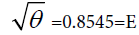1/G=SinE

1/G=1.5(MassGap)

1/G*G=1.0000

The Speed of Light

Here is why

c=2.9979~3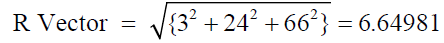Resistance

R=0.4233=cuz

E=0.8414=Sin1=Cos1

V=iR

0.8415/0.4233=198.8

66.4981/198.8=2.98949~3~c=speed of light

#### The Stable Universe and Free Will

Here is why the universe is stable and will last forever. It is also why humans have free will (Figures 5 and 6).

L=2(L/2+δ)

L=L/2Stress/Strain

L/2=F/(AE)

LAE=2(26.666)

LA=Volume=2F/R

125.999/19855=6.67=G

At G, the universe becomes a plastic hinge. We have free will. The Second Order Ode and The Taylor Series Solution [2-4].

The Solution to the Second Order Linear Ode is a Taylor Series That Gravitates Toward π –E. π-E Is Geometry Less Numbers To Base 10. Since Numbers are Simply Geometry in the Cartesian plane, Then We Have Geometry Less Geometry=0. This is a singularity. The Universe is therefore a Singularity. We See this in dE2/dt2 -E=Ln t=0 Ln t=0

Plastic Hinge Breaks Universe to Free Will Yet Minimizes Energy (Figure 7). Plastic Failure=De/Dt=0 Minimum Energy

div class="well well-sm">

#### Time Travel and the Singularity

At dE/dt=1

dE/dt=1 =Time Travel

y=etCos (2πt)

y'=et (1)

y=y'

et=1

t=Ln t=Ln 1=0

cos (2πt)=1

t=cos-1(1)/ (2π=0.5403/ 2π=0.086 =E/10

dE/dt=0.86/0.086=10

R=4.26

R2/2=4.26R

R=8.52

58.47°~59°

1/R=0.2018/0.86=0.1292

1/X=0.1292

Ln R=0.1292

R=2.298~23=prime

From the above estimation it can be stated that universe Gravitates Toward Opposite Poles.

#### A Unique Solution to the Equations Which Govern our Universe

If there existed anything at all, including space, then the Universe would necessarily exist exactly the way it does. Nothing is left to chance including me typing and you reading. Energy is what exists. And time is Energy stretched. Space is the cross product of Energy and time [5-7].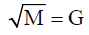E x t = ||E|| ||t|| sinθ

R2=[(1 x 8 x 22) *3]2=12

12=π* t*sin 57.29

t=4.539

1/t=0.2203

2*0.2203=0.4406

44.06/44=100.14

100.14(1/25)=1=E

So what n is 34=81=c4

1/81=0.012345679

Now,

t=M/c2

t=1/c2 *M

t=1/ (1+t)N N=11

1/c2=1/(1+t)11

#### The Universal Compounding Formula

(1+t)11=M/t

And, E=Mc2

M c2/c4=t=1/T=1/Period

π/100.14=31.8=Human Perception

The differential equation that summarizes our universe. linear homogenous differential equation with constant coefficients [8,9].

y=c1 *e(λ1x)+c2 e(λ2x)

=3*e1+3e(1)

=6e1=16.30

1/1.630=0.613

From above:

=(0.8415)(0.8415)(0.8415)

=0.6133

y=kcosh (λ1)t+ k sinh (λ2) t

#### General Solution

0.8415=k cosh (1)(t) + k sinh(1) (t)

0.8415/k=1.5431+1.1752

k=3.02 ~3 speed of light c

T=2.54~2.53

The plot of Energy Vs time converges at t=0.7 mathematics

1.7=0.1420 1-0.1420=0.858

Eigen Vector = 2.2467=t

Let t=1 E=y=e0.224 Cos (2π*t)=1.2514 =-E

Euler: c=3,

t=0.224=Eigenvector

1/t=Mass M=4.486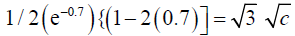The universe is simply described by 11 equations that break down into 4 equations. They are

P=Mv=F=Ma

d=vit +1/2 at2

Sqrt c/E=F=P

Time t is the Eigen vector. As energy rotates relative to it, there comes a point at 60° where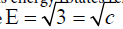. That is the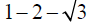triangle .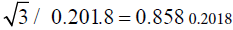is the initial condition for the dampened cosine curve y=et [Cos(2πt)]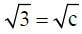F=P with M constant v=a=0.8415

It is simply modeled by waling up an up going escalator. Half the distance is due to velocity; the other half by acceleration. And that is the simplest way to understand our material universe.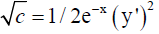Laplace =Euler

The Ultimate Universal Equation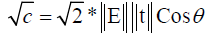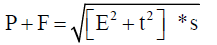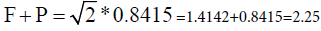M=2,25/1.73=13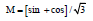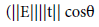1.73=Sqrt 2 *s

P+F=R*s

0.8415+0.8416=[(1,1,1) + 2 eigen vectors)]*s

2 sin 1=[2 sin 1 +2 Eigen Vectors] *s

Solving,

2Sin1 (1-s)=(2 * Eigen vectors)*s

0.8(0.1334)/0.1334=Eigen Vector

Vectors F=P

So, if we imagine a plot of P and F, Eigen Function=1.73=P+F

Fn.=Ma+Mv

=M(v+a)

M=1

F=P=Eg. Fn=M(v+a)

Derivative

Eg fn.'=dM/dt(a+a')

1.73=dM/dt (C1+C2)=sin +cos

INTEGRATE

1.73=C3M=sin +cos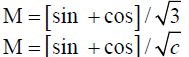So, Eg. Fn=sin +cos

Eg. Fn '=-cos +sin

Max=0

0=sin-cos

sin =cos

v=a

P=F

Finally,

Eg. fn=M(v+a)

Eg. fn'=dM/dt(v'+a')

0=a+a'

a=a'

y=y'

The Series solution to the ODE is a Singularity. It is 1/ R=1/0.4233=1/cuz.

1+0,84+1/2(0.842)(1)+1/6 (0.84)3(1)+1/24(0.84)4(1)=2.3143 =1/ cuz

y(0)=y'

1/R=y'

y=Ln R

y=Ln (2.3143)=0.8319~0.84=sin 1=cos 1

The material universe is the inverse of cuz. prime numbers and our universe

Difference

1, 1,2, 2, 4,2, 4,2,4

Or

1 1 2 2 22 2 22 2 22

2+210=211

(1+t)N

t=1

N=11

Now, Ln 211=7.6241

1/7.62=0.1312 or 0.868sin60°

Consider that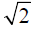is the minimum energy vector.

Ln 20.5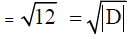And

FL=Moment=π

Ln 0,.2667 *Ln L=Lnπ

=0.2018=Minimum Energy=y=etcos (2πt)

Etsc *Ets=12

(1-cEts)=12

(1-3)(Ets)=12

-2 Ets=12

Ets=-12/2=6 cycles of time

dM/dt=2=Es

s=0.1334

Es=2=0.1334 (E)

E=15

1/E=t=0.666

Now M * dM/dt=c

4.486 (2)=8.972=c2

Integrate

M2/2 *M=s

M3=F=0.2668

M=0.6441

0.6441/4.486=7

1/7=0.1436 0.856

0.1334x=eπ

x Ln 0.1334=Ln eπ =π

x=0.0662~0.666

space = s = ||E||||t||cos 60=1/2

0.1334=1/2 y=0.2668=Force f

F/x=4.03 ~4=|D|

det |A- lambda I|=|D|

det |A-Lambda I| Pi=F

|D|=F/Geometry

det |A-Lambda I| Geometry =F

det |A-Lambda I| s=Ma

Units m2s2/kg

F L=N*m=Moment

So

det |A-Lambda I|=Moment

Moment *π =F

FL*π =F

L=1/π (31.8 Human Perception)

Moment *π =F

12*π =F

6*2π =F

6 cycles of time

sin 12π =0

cos 12π =1

So v=0 and a=1

d=vit+1/2 at2

0.134=0+1/2 (1)(1)^2

0.1334*2=F

d=Moment *π

π =E

Moment=d/E

E=s/Moment=0.1334/0.856=0.15-1-Moment

s/Moment=1-Moment

s/x=1-x

x/s=1/(1-x)

1/0.1334=1/(1-0.1334)

(1/7.49)-1 =1/1.1539=sin 60 degrees

#### Minimum Energy and the Singularity

The singularity is 1/x

From the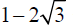triangle,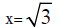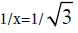=0.5774

3761BCE -1+2015 AD=5774 =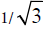t * Eigen Vector = 1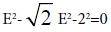E=2

E=1/t

t=1/E=1/2

Energy Parabola - Golden Mean

x2-x-1=0

(1/.2)2-1/2-1=0

-1.25=t

M/MT=7

s/M=7

1/7=M/s=E

E=M/s M=Es =0.856(0.1334)=0.1142

0.858

The only real numbers are prime numbers. We know 23 is the 10th prime number.

So Ln 23=3.1355~π

eπ =23.1406

y=y'

ex=1/x +C

x=t=π

C1=72.69 (72 rule)

E=dE/dt+dE/dt

E=2dE/dt

E2=4E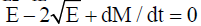dM/dt=2

(E-2)(E-2)=0

E=2

That's the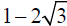triangle with t=1 and E=2

So π =1, E=2π =1 cycle.

eπ =1/π

ex=Ln x

eπ=Lnπ

Derivative

eπ =1/π +C1

C1=0.1359

0.8641 or 60°

As the Energy Vector rotates contraclockwise from the eigenvector t=1, when Resultant E=0.86, E=4.02 = |D|=FL

So t*FL =E and t=1,

The universe occurs at the singularity as illustrated above.

Ln π =eπ

1.1447=23.1406

23.1406/1.1447=0.1358

0.8642

60 degrees

e23.1406]/[Ln 23.1406=0.1358

0.8642

#### Conclusion

So here we presented the solution to the ODE’s that govern our universe. The other variables including Mass, Time, space and the Singularity and prime numbers are provided as to why they are important in our stable universe.

#### References

Select your language of interest to view the total content in your interested language

### Article Usage

• Total views: 8965
• [From(publication date):
September-2016 - Feb 26, 2020]
• Breakdown by view type
• HTML page views : 8836Can't read the image? click here to refresh